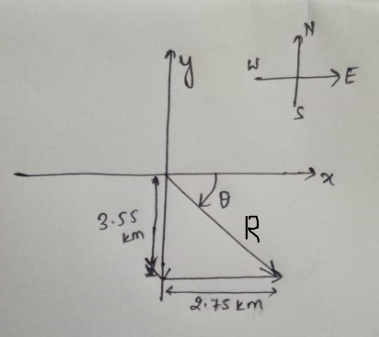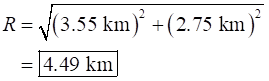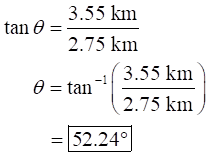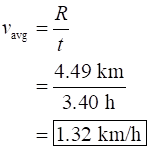# A man walks 3.55 km south and then 2.75 km east, all in 3.40 hours.(a)What is the magnitude (in km) and direction (in degrees south of east) of his displacement during the given time?magnitude km direction ° south of east(b)What is the magnitude (in km/h) and direction (in degrees south of east) of his average velocity during the given time?magnitude km/h direction ° south of east(c)What was his average speed (in km/h) during the same time interval?km/h

Question
4 views
A man walks 3.55 km south and then 2.75 km east, all in 3.40 hours.
(a)
What is the magnitude (in km) and direction (in degrees south of east) of his displacement during the given time?
magnitude km direction ° south of east
(b)
What is the magnitude (in km/h) and direction (in degrees south of east) of his average velocity during the given time?
magnitude km/h direction ° south of east
(c)
What was his average speed (in km/h) during the same time interval?
km/h

check_circle

Step 1

Draw the position diagram for the man as shown below.Step 2

(a)

Using position diagram and Pythagoreans theorem the magnitude of the displacement is:Use the trigonometrical identity and determine the direction of the displacement as:Therefore, the magnitude of the displacement during the given time is 4.49 km and the direction is 52.24 south of east.

Step 3

(b)

The formula for the average velocity is:The direction of the average velocity is same as the direction of the displacement.

Therefore, the average velocity of the man is 1.32 km/h direction of the velocity is 52.24 south of east.

...

### Want to see the full answer?

See Solution

#### Want to see this answer and more?

Solutions are written by subject experts who are available 24/7. Questions are typically answered within 1 hour.*

See Solution
*Response times may vary by subject and question.
Tagged in